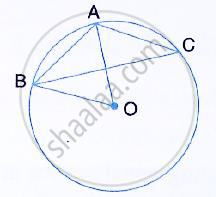Share

In the Given Figure, Ab is a Side of a Regular Six-sided Polygon and Ac is a Side of a Regular Eight Sided Polygon Inscribed in the Circle with Centre O. Calculate the Sizes Of: (I) ∠Aob, - ICSE Class 10 - Mathematics

Question

In the given figure, AB is a side of a regular six-sided polygon and AC is a side of a regular eight sided polygon inscribed in the circle with centre O. Calculate the sizes of:
(i) ∠AOB,  (ii) ∠ACB  (iii) ∠ABCSolution

(i) Arc AB subtends ∠AOB at the centre and
∠ACB at the remaining part of the circle.
∴ ∠ACB = 1/2∠AOB
Since AB is the side of a regular hexagon,
∠AOB = 60°

(ii) ∠AOB = 60° ⇒ ∠ACB = 1/2 xx60° = 30°

(iii) Since AC is the side of a regulare octagon,
∠AOC = (360°) /8= 45°

Again, Arc AC subtends ∠AOC at the center and
∠ABC at the remaining part of the circle.
⇒ ∠ABC = 1/2 ∠AOC

⇒ ∠ABC = (45°)/2 = 22.5°

Is there an error in this question or solution?
Solution In the Given Figure, Ab is a Side of a Regular Six-sided Polygon and Ac is a Side of a Regular Eight Sided Polygon Inscribed in the Circle with Centre O. Calculate the Sizes Of: (I) ∠Aob, Concept: Concept of Circles.
S